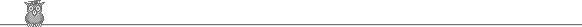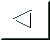## Automatic validation of numerical solutions

### Ole Stauning

For a copy of this publication, either

## Abstract

This thesis is concerned with ``Automatic Validation of Numerical Solutions''. The basic theory of interval analysis and self-validating methods is introduced. The mean value enclosure is applied to discrete mappings for obtaining narrow enclosures of the iterates when applying these mappings with intervals as initial values. A modification of the mean value enclosure of discrete mappings is considered, namely the extended mean value enclosure which in most cases leads to even better enclosures. These methods have previously been described in connection with discretizing solutions of ordinary differential equations, but in this thesis, we describe how to use the methods for enclosing iterates of discrete mappings, and then later use them for discretizing solutions of ordinary differential equations.

The theory of automatic differentiation is introduced, and three methods for obtaining derivatives are described: The forward, the backward, and the Taylor expansion methods. The three methods have been implemented in the C++ program packages FADBAD/TADIFF. Some examples showing how to use the three metho ds are presented. A feature of FADBAD/TADIFF not present in other automatic differentiation packages is the possiblility to combine the three methods in an extremely flexible way. We examine some applications where this flexibility is very useful.

A method for Taylor expanding solutions of ordinary differential equations is presented, and a method for obtaining interval enclosures of the truncation errors incurred, when truncating these Taylor series expansions is described. By combining the forward method and the Taylor expansion method, it is possible to implement the (extended) mean value enclosure of a truncated Taylor series expansion with enclosures of the truncation errors. A C++ program package ADIODES, using this method has been developed. (ADIODES is an abbreviation of `` Automatic Differentiation Interval Ordinary Differential Equation Solver'').

ADIODES is used to prove existence and uniqueness of periodic solutions to specific ordinary differential equations occuring in dynamical systems theory. These proofs of existence and uniqueness are difficult or impossible to obtain using other known methods. Also, a method for solving boundary value problems is described.

Finally a method for enclosing solutions to a class of integral equations is described. This method is based on the mean value enclosure of an integral operator and uses interval Bernstein polynomials for enclosing the solution. Two numerical examples are given, using two orders of approximation and using different numbers of discretization points.

## IMM ph.d thesis 36, 1997Go back